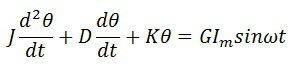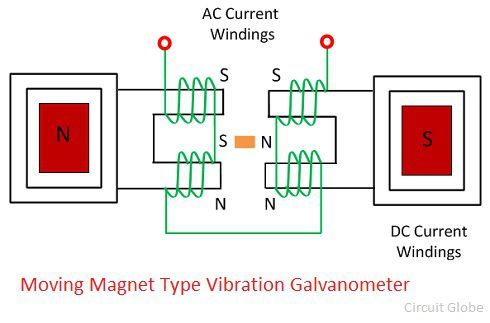# Vibration Galvanometer

Definition: The galvanometer in which the oscillation frequency of the moving element and the measurand current becomes equal is known as the vibration galvanometer. It uses for detecting the alternating current or alternating electromotive force.

## Working Principle of Vibration Galvanometer

When an alternating current is passing through the moving element of the galvanometer, deflecting torque produces because of which the coil vibrates. If the vibration frequency of the moving element is equal to the frequency of the measurand current, the moving element vibrates with large amplitude.

## Types of Vibration Galvanometer

The vibration galvanometer are of two types

1. Moving Iron Type Vibration Galvanometer
2. Moving Magnet Type Vibration Galvanometer

### Moving Iron Type Vibration Galvanometer

In moving coil type instrument, the coil suspends between the poles of the permanent magnet. The measurand AC when passes through the moving coil produce the deflection torque on it.

### Construction of Vibration Galvanometer

In vibration galvanometer, the moving element is suspended between the gap of a permanent magnet or in the field of an electromagnet. The moving element suspends with the help of fine bronze or platinum wire. The wire is attached to the pulley at the top. The pulley with the help of the spring keeps the string or wire tight.

The wire or string stretches between the two ivory bridges, the position of the bridges can be adjusted according to the requirement. The tension on the spring depends on the position of the ivory bridges. The mirror is placed on the string or thread between the ivory bridges. When the measurand current passes through the coil, the reflected beam through the mirror focus on the scale.The natural oscillation frequency of the moving element is tuned to the specified frequency. The tunning is the adjustment of the natural frequency oscillation so that it is equal to the frequency of measurand current passing through it.

The tuning of the vibration galvanometer depends on the tension of the suspension spring. The tension on the spring means the pulling force acts axially on the spring. The tuning increases the amplitude of vibration because of which the wide band of light is reflected on the screen.

### Theory of Vibration Galvanometer

Let, i be the current passing through the coil of a vibration galvanometer.The equation of motion is also expressed asWhere, G – Displacement Constant
J – Constant of Inertia
D – Damping Constant
K – Control Constant
θ – Angle of deflection

Note – The amplitude of the vibration galvanometer increased by increasing the damping constant G. The constant damping increases by using the coil of the large area along with the maximum number of turns.

### Moving Magnet Type Vibration Galvanometer

The operation of the moving magnet type vibration galvanometer depends on the magnetic field of the resonance frequency. The resonance is the phenomenon in which the one oscillating system oscillates the other system with the great amplitude at the fixed frequency. The frequency at which the relative amplitude becomes maximum is known as the response frequency.In moving magnet type instrument the small piece of magnet is suspended between the poles of two permanent magnets along with the mirror. The measurand current passes through the coil because of which the magnetic field develops around them.

The small magnetic field starts rotating between the poles of a magnet. The electromagnets are also used in place of the permanent magnets. The electromagnet energises through the measurand current passes through the coil.

In moving magnet type vibration galvanometer, the tuning between the moving system and the frequency can be done by adjusting the tension or suspension of the spring and the magnetic field between the pole of magnets.